# Magnetism slides

5. Aug 2010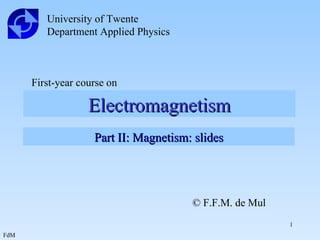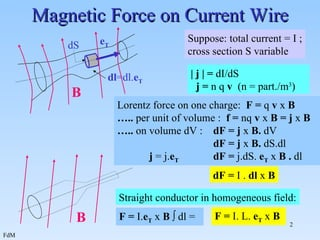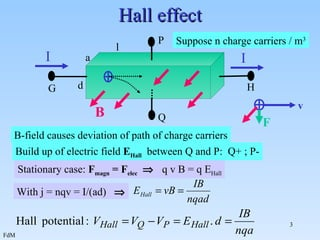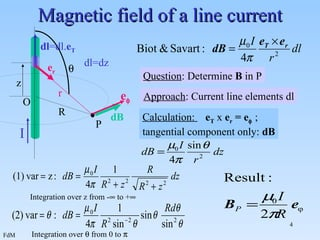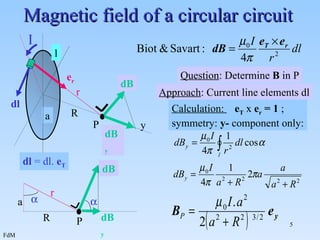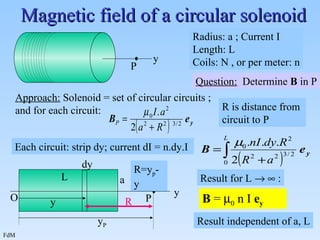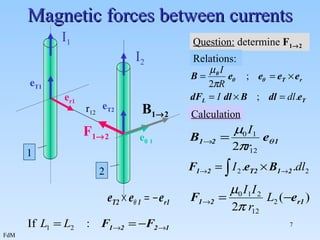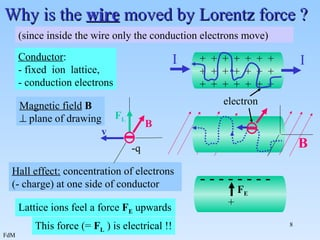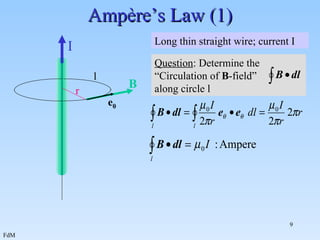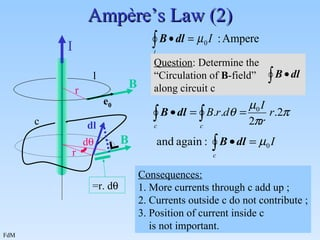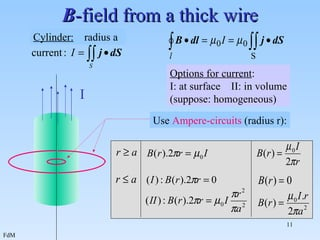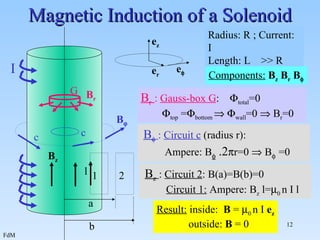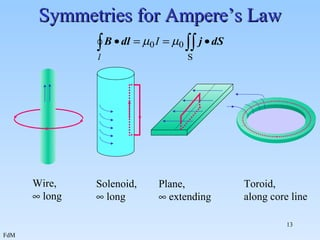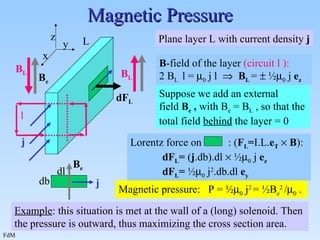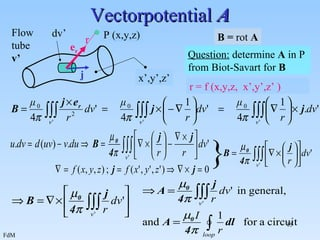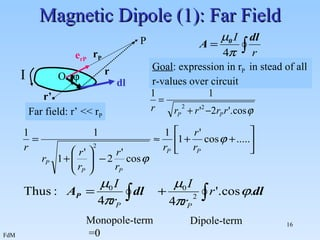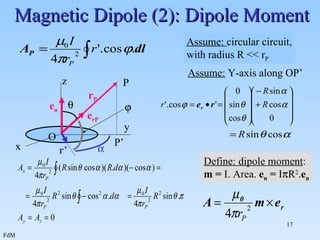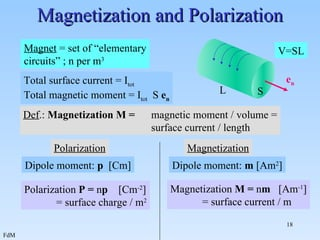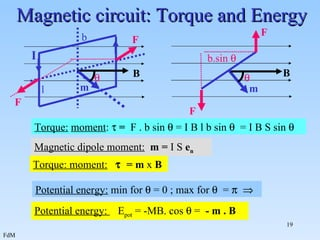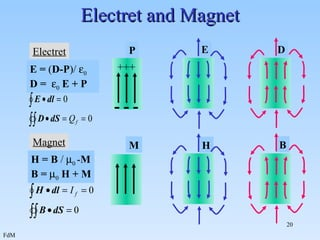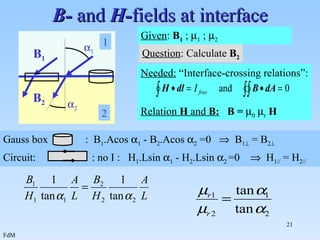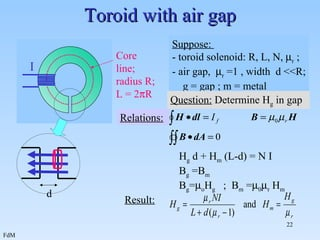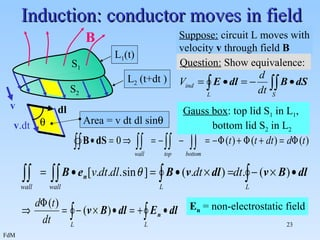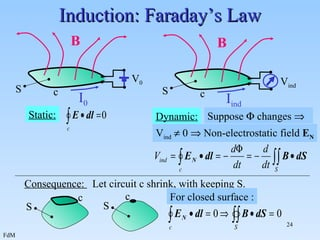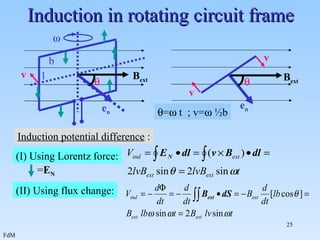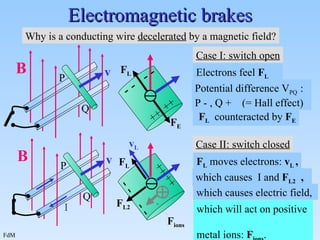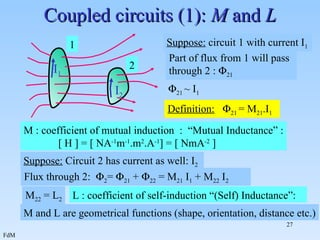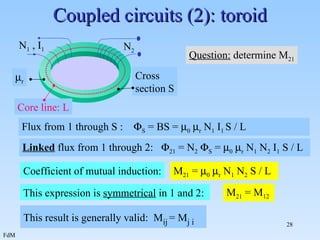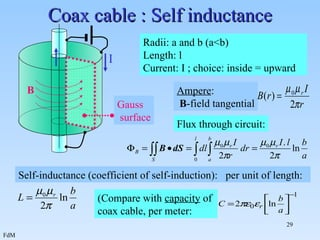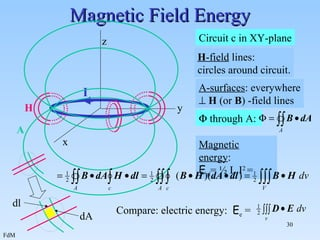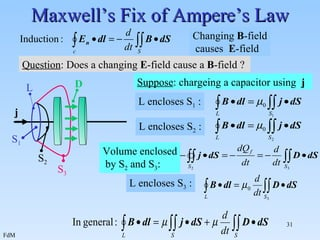1 von 31

### Magnetism slides

• 1. Electromagnetism University of Twente Department Applied Physics First-year course on Part II: Magnetism: slides © F.F.M. de Mul
• 2. Magnetic Force on Current Wire Lorentz force on one charge: F = q v x B … .. per unit of volume : f = nq v x B = j x B … .. on volume dV : dF = j x B. dV dF = j x B. dS.dl j = j. e T dF = j.dS. e T x B . dl dF = I . dl x B F = I. e T x B  dl = F = I. L. e T x B e T dS dl =dl. e T | j | = dI/dS j = n q v (n = part./m 3 ) Suppose: total current = I ; cross section S variable B Straight conductor in homogeneous field: B
• 3. Hall effect B-field causes deviation of path of charge carriers Stationary case: F magn = F elec  q v B = q E Hall Build up of electric field E Hall between Q and P: Q+ ; P- With j = nqv = I/ ( ad )  G H P Q B I I d l a Suppose n charge carriers / m 3 F v
• 4. Magnetic field of a line current Integration over z from -  to +  Integration over  from 0 to  I P R Question : Determine B in P O dl=dz  e r Approach : Current line elements dl dl =dl. e T z r Calculation: e T x e r = e  ; tangential component only: dB dB e 
• 5. Magnetic field of a circular circuit Question : Determine B in P P R y I l a Approach : Current line elements dl r e r dl dl = dl. e T R r a dB P   dB y Calculation: e T x e r = 1 ; symmetry: y- component only: dB dB y
• 6. Magnetic field of a circular solenoid Each circuit: strip dy; current dI = n.dy.I Result independent of a, L Radius: a ; Current I Length: L Coils: N , or per meter: n Approach: Solenoid = set of circular circuits ; and for each circuit: R is distance from circuit to P Question: Determine B in P y P Result for L   : B =  0 n I e y P O y y P L a dy y R=y p -y R
• 7. Magnetic forces between currents Question: determine F 1  2 1 2 I 1 I 2 r 12 e T1 e T2 e r1 e  1 Relations: F 1  2 B 1  2 Calculation
• 8. Why is the wire moved by Lorentz force ? (since inside the wire only the conduction electrons move) This force (= F L ) is electrical !! + + + + + + + + + + + + + + + + + + + + + I I electron Conductor : - fixed ion lattice, - conduction electrons Hall effect: concentration of electrons (- charge) at one side of conductor - - - - - - - - Lattice ions feel a force F E upwards + F E B v F L -q B Magnetic field B  plane of drawing
• 9. Ampère’s Law (1) I Long thin straight wire; current I B e  r Question : Determine the “ Circulation of B -field” along circle l l
• 10. Ampère’s Law (2) I B e  r l Consequences: 1. More currents through c add up ; 2. Currents outside c do not contribute ; 3. Position of current inside c is not important. Question : Determine the “ Circulation of B -field” along circuit c c r B dl =r. d  d 
• 11. B -field from a thick wire Options for current : I: at surface II: in volume (suppose: homogeneous) I Cylinder: radius a Use Ampere-circuits (radius r):
• 12. Magnetic Induction of a Solenoid Result: inside: B =  0 n I e z outside: B = 0 Radius: R ; Current: I Length: L >> R Coils: n per meter I e z e r e  Components: B z B r B  G B r : Gauss-box G :  total =0  top =  bottom   wall =0  B r =0 B r c c B  : Circuit c (radius r): Ampere: B  .2  r=0  B  =0 B  1 2 B z : Circuit 2 : B(a)=B(b)=0 Circuit 1: Ampere: B z l=  0 n I l a b l B z
• 13. Symmetries for Ampere’s Law Wire,  long Plane,  extending Solenoid,  long Toroid, along core line
• 14. Magnetic Pressure Magnetic pressure: P = ½  0 j 2 = ½B e 2 /  0 . Example : this situation is met at the wall of a (long) solenoid. Then the pressure is outward, thus maximizing the cross section area. Plane layer L with current density j j L Suppose we add an external field B e , with B e = B L , so that the total field behind the layer = 0 B e j Lorentz force on : ( F L = I.L. e T  B ): dF L = ( j .db).dl  ½  0 j e z dF L = ½  0 j 2 .db.dl e y dl db B e dF L B -field of the layer (circuit l ): 2 B L l =  0 j l  B L =  ½  0 j e z l z B L B L x y
• 15. Vectorpotential A r = f (x,y,z, x’,y’,z’ ) } e r P (x,y,z) r d v ’ Flow tube v’ x’,y’,z’ B = rot A Question: determine A in P from Biot-Savart for B j
• 16. Magnetic Dipole (1): Far Field Far field: r’ << r P Monopole-term =0 Dipole-term Goal : expression in r P in stead of all r-values over circuit r P r r’ O e rP dl P I 
• 17. Magnetic Dipole (2): Dipole Moment Assume: Y-axis along OP’ Assume: circular circuit, with radius R << r P P P’ y x z   r’ e rP r P O  Define: dipole moment : m = I. Area. e n = I  R 2 . e n e n
• 18. Magnetization and Polarization Total surface current = I tot Total magnetic moment = I tot S e n L S e n Magnet = set of “elementary circuits” ; n per m 3 Polarization Magnetization Dipole moment: p [Cm] Dipole moment: m [Am 2 ] Polarization P = n p [Cm -2 ] = surface charge / m 2 Magnetization M = n m [Am -1 ] = surface current / m Def .: Magnetization M = magnetic moment / volume = surface current / length V=SL
• 19. Magnetic circuit: T orque and Energy Torque: moment :  = F . b sin  = I B l b sin  = I B S sin  Magnetic dipole moment: m = I S e n Torque: moment:  = m x B Potential energy: min for  = 0 ; max for  =   Potential energy: E pot = -MB. cos  = - m . B B m F F F F m B b l b.sin    I
• 20. Electret and Magnet E D H B Electret P - - - +++ E = ( D-P )/  0 D =  0 E + P Magnet M H = B /  0 - M B =  0 H + M
• 21. B- and H- fields at interface Given : B 1 ;  1 ;  2 Question : Calculate B 2 B 1 B 2  1  2 1 2 Gauss box : B 1 .Acos  1 - B 2 .Acos  2 =0  B 1  = B 2  Circuit: : no I : H 1 .Lsin  1 - H 2 .Lsin  2 =0  H 1// = H 2// Needed: “Interface-crossing relations”: Relation H and B: B =  0  r H
• 22. Toroid with air gap Question: Determine H g in gap H g d + H m (L-d) = N I B g =B m B g =  o H g ; B m =  0  r H m Suppose: - toroid solenoid: R, L, N,  r ; - air gap,  r =1 , width d <<R; g = gap ; m = metal I Core line; radius R; L = 2  R Relations: Result: d
• 23. Induction: conductor moves in field E n = non-electrostatic field B v L 1 (t) L 2 (t+dt ) Suppose: circuit L moves with velocity v through field B Question: Show equivalence: v .dt dl  Area = v dt dl sin  Gauss box : top lid S 1 in L 1 , bottom lid S 2 in L 2 S 1 S 2
• 24. Induction: Faraday’s Law Suppose  changes  V ind  0  Non-electrostatic field E N V 0 B c S I 0 Static: V ind Dynamic: B c S I ind Consequence: Let circuit c shrink, with keeping S. S c c S For closed surface :
• 25. Induction in rotating circuit frame Induction potential difference : (II) Using flux change: B ext e n B ext b l   v v v e n   =  t ; v=  ½b (I) Using Lorentz force: = E N
• 26. Electromagnetic brakes Why is a conducting wire decelerated by a magnetic field? Case I: switch open P Q v B Electrons feel F L F L P Q v B Case II: switch closed Potential difference V PQ : P - , Q + (= Hall effect) ++ ++ - - - - F L v L F L moves electrons: v L , which will act on positive metal ions: F ions . F ions F E F L counteracted by F E I F L2 which causes I and F L2 , ++ ++ - - - - which causes electric field,
• 27. Coupled circuits (1): M and L Flux through 2:  2 =  21 +  22 = M 21 I 1 + M 22 I 2 M and L are geometrical functions (shape, orientation, distance etc.) 1 2 I 1 Suppose: circuit 1 with current I 1 Part of flux from 1 will pass through 2 :  21  21 ~ I 1 Definition:  21 = M 21 .I 1 M : coefficient of mutual induction : “Mutual Inductance” : [ H ] = [ NA -1 m -1 .m 2 .A -1 ] = [ NmA -2 ] Suppose: Circuit 2 has current as well: I 2 I 2 L : coefficient of self-induction “(Self) Inductance”: M 22 = L 2
• 28. Coupled circuits (2): toroid Question: determine M 21 Flux from 1 through S :  S = BS =  0  r N 1 I 1 S / L Linked flux from 1 through 2:  21 = N 2  S =  0  r N 1 N 2 I 1 S / L This result is generally valid: M ij = M j i Cross section S N 1 , I 1 N 2 Core line: L  r Coefficient of mutual induction: M 21 =  0  r N 1 N 2 S / L This expression is symmetrical in 1 and 2: M 21 = M 12
• 29. Coax cable : Self inductance Flux through circuit: Radii: a and b (a<b) Length: l Current: I ; choice: inside = upward I Ampere : B -field tangential Self-inductance (coefficient of self-induction): per unit of length: (Compare with capacity of coax cable, per meter: Gauss surface B
• 30. Magnetic Field Energy Magnetic energy : E m = ½ L I 2 = x z y I Circuit c in XY-plane H H -field lines: circles around circuit. A A-surfaces : everywhere  H (or B ) -field lines  through A: dA dl Compare: electric energy: E e =
• 31. Maxwell’s Fix of Ampere’s Law Changing B -field causes E -field Question : Does a changing E -field cause a B -field ? j Suppose : chargeing a capacitor using j L S 1 L encloses S 1 : S 2 L encloses S 2 : L encloses S 3 : S 3 Volume enclosed by S 2 and S 3 : D